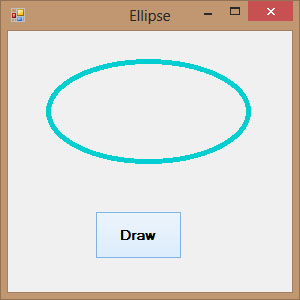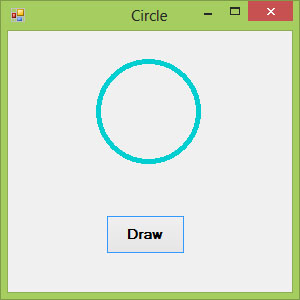# Visual Basic 2013 Lesson 27: Drawing Ellipse and Circle

#### [Lesson 26] << [Contents] >> [Lesson 28]

We have learned how to draw rectangles with various line styles in Visual Basic 2013 in the previous lesson. In this lesson, we shall learn how to draw ellipse and circle. As a matter of fact, The method to draw an ellipse and circle is the same.

### 27.1 Drawing an Ellipse

First of all, we need to understand the principle behind drawing an ellipse in Visual Basic 2013. The basic structure of most shapes is a rectangle, an ellipse is no exception. Ellipse is an oval shape that is bounded by a rectangle, as shown in Figure 27.1Figure 27.1

Therefore, we need to create a Rectangle object before we can draw an ellipse. This rectangle serves as a bounding rectangle for the ellipse. However, you still need to use the DrawEllipse method to finish the job. On the other hand, we can also draw an ellipse with the DrawEllipse method without first creating a rectangle. We shall show you both methods. In the first method, let’s say you have created a rectangle object known as myRectangle and a pen object as myPen, then you can draw an ellipse using the following statement:

`myGraphics.DrawEllipse(myPen, myRectangle)`

* Assume you have also already created the Graphics object myGraphics.

#### Example 27.1(a)

```Private Sub BtnDraw_Click(sender As Object, e As EventArgs) Handles BtnDraw.Click
Dim myPen As Pen
myPen = New Pen(Drawing.Color.DarkTurquoise, 5)
Dim myGraphics As Graphics = Me.CreateGraphics
Dim myRectangle As New Rectangle
myRectangle.X = 40
myRectangle.Y = 30
myRectangle.Width = 200
myRectangle.Height = 100
myGraphics.DrawEllipse(myPen, myRectangle)
End Sub
```

The output image is shown in Figure 27.2Figure 27.2

The second method is using the DrawEllipse method without creating a rectangle object. Of course, you still have to create the Graphics and the Pen objects. The syntax is:

```myGraphics.DrawEllipse(myPen, X,Y,Width, Height)
```

Where (X, Y) are the coordinates of the upper-left corner of the bounding rectangle, width is the width of the ellipse and height is the height of the ellipse.

#### Example 27.1(b)

```Private Sub BtnDraw_Click(sender As Object, e As EventArgs) Handles BtnDraw.ClickDim myPen As Pen
myPen = New Pen(Drawing.Color.DarkTurquoise, 5)
Dim myGraphics As Graphics = Me.CreateGraphics
myGraphics.DrawEllipse(myPen, 40, 30, 200, 100)
End Sub
```

### 27.2 Drawing a Circle

After you have learned how to draw an ellipse, drawing a circle becomes very simple. We use exactly the same methods used in the preceding section but modify the width and height so that they are of the same values.

The following examples draw the same circle.

Example 27.2(a)

```Dim myPen As Pen
myPen = New Pen(Drawing.Color.DarkTurquoise, 5)
Dim myGraphics As Graphics = Me.CreateGraphics
Dim myRectangle As New Rectangle
myRectangle.X = 90
myRectangle.Y = 30
myRectangle.Width = 100
myRectangle.Height = 100
myGraphics.DrawEllipse(myPen, myRectangle)
```

#### Example 27.2(b)

```Dim myPen As Pen
myPen = New Pen(Drawing.Color.DarkTurquoise, 5)
Dim myGraphics As Graphics = Me.CreateGraphics
myGraphics.DrawEllipse(myPen, 90, 30, 100, 100)
```

The output image is shown in Figure 27.3Figure 27.3